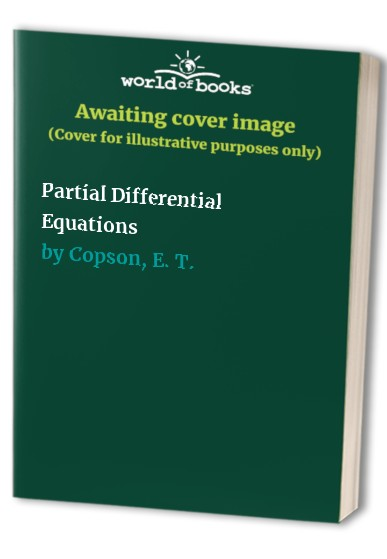# Partial Differential Equations by E. T. Copson

In Stock
\$33.99
+10% GST
In this book, Professor Copson gives a rigorous account of the theory of partial differential equations of the first order
Only 1 left

## Partial Differential Equations Summary

### Partial Differential Equations by E. T. Copson

In this book, Professor Copson gives a rigorous account of the theory of partial differential equations of the first order and of linear partial differential equations of the second order, using the methods of classical analysis. In spite of the advent of computers and the applications of the methods of functional analysis to the theory of partial differential equations, the classical theory retains its relevance in several important respects. Many branches of classical analysing have their origins in the rigourous discussion of problems in applies mathematics and theoretical physics, and the classical treatment of the theory of partial differential equations still provides the best method of treating many physical problems. A knowledge of the classical theory is essential for pure mathematics who intend to undertake research in this field, whatever approach they ultimately adopt. The numerical analyst needs a knowledge of classical theory in order to decide whether a problem has a unique solution or not.

### Why buy from World of BooksOur excellent value books literally don't cost the earthFree delivery in AustraliaEvery used book bought is one saved from landfill

Preface; 1. Partial differential equations of the first order; 2. Characteristics of equations of the second order; 3. Boundary value and initial value problems; 4. Equations of hyperbolic type; 5. Reimann's method; 6. The equation of wave motions; 7. Marcel Riesz's method; 8. Potential theory in the plane; 9. Subharmonic functions and the problem of Dirichlet; 10. Equations of elliptic type in the plane; 11. Equations of elliptic type in space; 12. The equation of heat; Appendix; Books for further reading; Index.

GOR009720357
Partial Differential Equations by E. T. Copson
E. T. Copson
Used - Very Good
Hardback
Cambridge University Press
1975-10-02
287
0521205832
9780521205832
N/A
Book picture is for illustrative purposes only, actual binding, cover or edition may vary.
This is a used book - there is no escaping the fact it has been read by someone else and it will show signs of wear and previous use. Overall we expect it to be in very good condition, but if you are not entirely satisfied please get in touch with us.